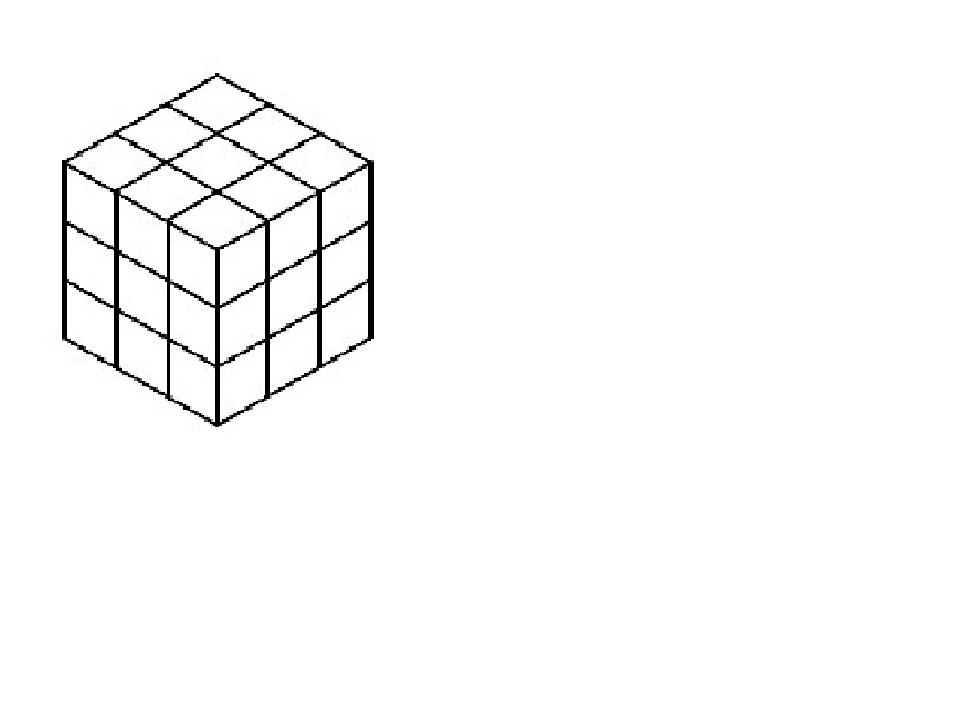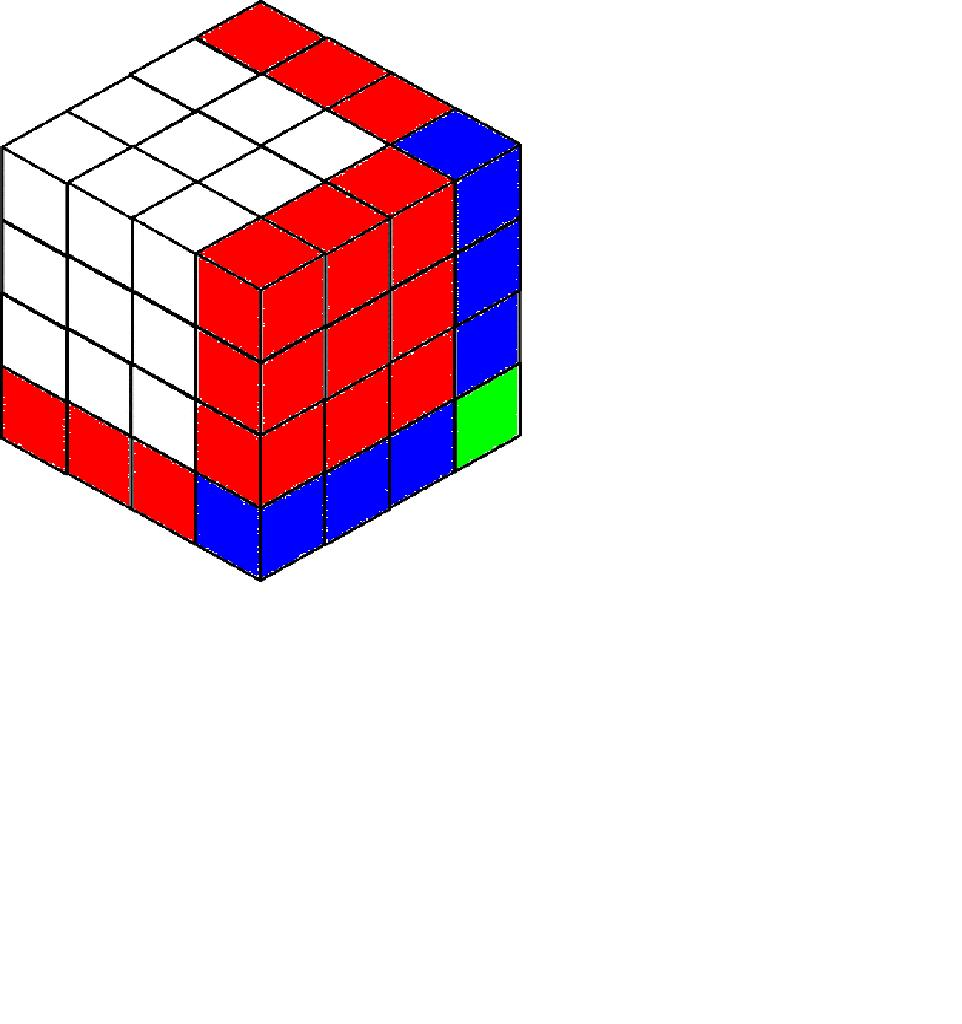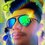# Judge this proof: Fermat's last theorem for n=3

So first of all the equation, $(a^{3}+b^{3}=c^{3})$ for which I thick i can prove there is no whole number solution for it. At the end you can know why I can do it only for 3 (because of the method I used).

Proof by contradiction.

So, as $(a+b)^{3}=a^{3}+b^{3}+3a^{2}b+3ab^{2}$

$a^{3}+b^{3}=(a+b)^{3}-3ab(a+b)$

$c^{3}=(a+b)^{3}-3ab(a+b)$

$c=[(a+b)^{3}-3ab(a+b)]^{\frac{1}{3}}$

So now for $"c"$ to be an whole number, the term $(a+b)^{3}-3ab(a+b)$ must be a cube

Now let us think about the term $3ab(a+b)$ and what will be its form so that its subtraction will lead to a cube.

• Imagine a (4 by 4) cube to turn it into (3 by 3)• there are 64 small cubes in 4 by 4
• there are 27 small cubes in 3 by 3
• to convert it will be $4^{3}-x=3^{3}$
• here the $x$ has form ${\color{#D61F06} [3\cdot 1\cdot (4)^{2}]}-{\color{#3D99F6} [3\cdot 1^{2}\cdot 4]}+{\color{#20A900} ^{3}}$

• Now similarly we convert $(a+b)^{3}$ into any other cube by subtracting $3ab(a+b)$
• With above logic we note that $3ab(a+b)$ must be of form,
• $3ab(a+b)=3k(a+b)^{2}-3k^{2}(a+b)+k^{3}$, where $k$ is a natural number [IMP step]
• so now we prove that this equation is cannot be true(and thus contradiction)
• $3k(a+b)[a+b-k]+k^{3}=3ab(a+b)$
• by inspecting we conclude that $k$ is multiple of 3 so let us assume that$k=3m$

$\Rightarrow 9m(a+b)[a+b-3m]+27m^{3}=3ab(a+b)$

$\Rightarrow 3m(a+b)[a+b-3m]+9m^{3}=ab(a+b)$

$\Rightarrow 3m(a+b)^{2}-9m^{2}(a+b)+9m^{3}=ab(a+b)$

$\Rightarrow 3m(a+b)-9m^{2}+\frac{9m^{3}}{a+b}=ab$

$\Rightarrow$ we conclude that $m=(a+b)n$ and thus $k=3(a+b)n$,where $n$ is natural number.

$\Rightarrow 3(a+b)^{2}n-9n^{2}(a+b)^{2}+9n^{3}(a+b)^{2}=ab$

$\Rightarrow (a+b)^{2}[3n+9n^{3}-9n^{2}]=ab$

$\Rightarrow$ Let $[3n+9n^{3}-9n^{2}]$ be $S$

$\Rightarrow (a+b)^{2}S=ab$

$\Rightarrow Sa^{2}+Sb^{2}+2Sab=ab$

$\Rightarrow Sa^{2}+Sb^{2}+(2S-1)ab=0$

$\Rightarrow$ now note that $S$ is more than 3 for natural $n$ (not proving that)

$\Rightarrow$ so in $L.H.S.$ everything is positive and in $R.H.S.$ there is zero

$\Rightarrow$ so we conclude that there exist no natural $k$ for which $3ab(a+b)=3k(a+b)^{2}-3k^{2}(a+b)+k^{3}$ which is necessary condition for whole number solutions of $(a^{3}+b^{3}=c^{3})$.

$\Rightarrow$ so it is a $\boxed{\mathbf{CONTRADICTION}}$

The proof is incomplete, and it is in progress when $(a+b)$ doesn't divides $m$Note by Yash Ghaghada
3 years, 2 months ago

This discussion board is a place to discuss our Daily Challenges and the math and science related to those challenges. Explanations are more than just a solution — they should explain the steps and thinking strategies that you used to obtain the solution. Comments should further the discussion of math and science.

When posting on Brilliant:

• Use the emojis to react to an explanation, whether you're congratulating a job well done , or just really confused .
• Ask specific questions about the challenge or the steps in somebody's explanation. Well-posed questions can add a lot to the discussion, but posting "I don't understand!" doesn't help anyone.
• Try to contribute something new to the discussion, whether it is an extension, generalization or other idea related to the challenge.
• Stay on topic — we're all here to learn more about math and science, not to hear about your favorite get-rich-quick scheme or current world events.

MarkdownAppears as
*italics* or _italics_ italics
**bold** or __bold__ bold
- bulleted- list
• bulleted
• list
1. numbered2. list
1. numbered
2. list
Note: you must add a full line of space before and after lists for them to show up correctly
paragraph 1paragraph 2

paragraph 1

paragraph 2

[example link](https://brilliant.org)example link
> This is a quote
This is a quote
    # I indented these lines
# 4 spaces, and now they show
# up as a code block.

print "hello world"
# I indented these lines
# 4 spaces, and now they show
# up as a code block.

print "hello world"
MathAppears as
Remember to wrap math in $$ ... $$ or $ ... $ to ensure proper formatting.
2 \times 3 $2 \times 3$
2^{34} $2^{34}$
a_{i-1} $a_{i-1}$
\frac{2}{3} $\frac{2}{3}$
\sqrt{2} $\sqrt{2}$
\sum_{i=1}^3 $\sum_{i=1}^3$
\sin \theta $\sin \theta$
\boxed{123} $\boxed{123}$

## Comments

Sort by:

Top Newest

don't judge grammar and typos

- 3 years, 2 months ago

Log in to reply

also you can do similar thing for n=2 (square) but there you will end up with condition and not contradiction

- 3 years, 2 months ago

Log in to reply

The beginning is confusing and a little more complicated than it needs to be. You're writing $a^3+b^3=c^3,$ and letting $c=a+b-k.$ Expanding this leads to the "IMP step" $3ab(a+b) = 3k(a+b)^2-3k^2(a+b)+k^3.$ I agree that $k=3m$ for some $m,$ and that leads to $3m(a+b)-9m^2+\frac{9m^3}{a+b} = ab.$ (There's a typo in your version of this equation--you temporarily forgot to cancel $a+b$ from the right side, but that's corrected on the next line.)

The argument fails here: you claim that $a+b$ divides $m.$ It divides $9m^3,$ yes, but not necessarily $m.$ Your argument works when $a+b$ is a prime not equal to $3,$ but that case is easy from the original equation: $(a+b)(a^2-ab+b^2) = c^3,$ so $p=a+b$ divides $c^3,$ hence $c,$ so $p|(a^2-ab+b^2),$ but it also divides $p^2 = a^2+2ab+b^2,$ so $p|3ab,$ but it can't divide $3,a,b,$ done.

- 3 years, 2 months ago

Log in to reply

Hi, I appreciate for your point of view but I don't get what you are saying actually ( why c=a+b-k) . And thanks for the typo

- 3 years, 2 months ago

Log in to reply

Don't worry about that part, I'm just re-deriving your formula more simply and quickly. The important part of the post is where I point out that the argument fails (the "we conclude that..." statement is not necessarily true).

- 3 years, 2 months ago

Log in to reply

but why because $m$ is some natural number $n$ is some natural number

e.g $(a+b)=7$ and if $n=1$ then $m=7$ and $k=21$

if $n=2$ then $m=14$ and $k=42$ and so on.....

e.g $(a+b)=6$ and if $n=1$ then $m=6$ and $k=18$

if $n=2$ then $m=12$ and $k=36$ and so on.....

- 3 years, 2 months ago

Log in to reply

The problem is that $n$ is not necessarily a natural number: $\frac{9m^3}{a+b}$ is an integer, but it's not necessarily true that $\frac{m}{a+b}$ is an integer. For instance $a+b=12, m =2.$

- 3 years, 2 months ago

Log in to reply

ok ok I get it. but there are only 3 cases i.e

$a+b=3$

$a+b=9$

or $m=(a+b)n$ correct me I am wrong

- 3 years, 2 months ago

Log in to reply

No, this is still wrong. Again, look at my example, where $a+b=12.$ There are infinitely many counterexamples. It’s a serious hole.

- 3 years, 2 months ago

Log in to reply

oh I get it, but my idea is correct that the equation is not true. I will update the note as I figure out to solve that problem and thank you

- 3 years, 2 months ago

Log in to reply

Watch Mathologer's proof of Fermat's Last Theorem.

- 2 years, 8 months ago

Log in to reply

×

Problem Loading...

Note Loading...

Set Loading...